### Localizations of a ring at specific elements correspond to sections of a sheaf

One fundamental observation in algebraic geometry is that there are a number of powerful correspondences between ring localizations and algebraic sheaves. Although elementary, this post will outline one such correspondence, which is that the localizations of a ring to a specific element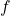can be exactly thought of as the sections of the sheaf of regular functions over the distinguished open set of. (Consider this post to be a self-directed refresher! It has been a long time since I learned this material.)

Suppose that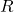is a finitely generated algebra over an algebraically closed field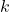. One might naturally wonder if there is any geometric interpretation of the localization of this ring at a single one of its elements. Say we take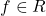(where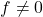) and consider the local ring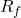, i.e. quotients of the form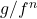for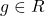and nonnegative integers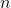. If we considerand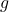as polynomials to be evaluated, then these quotients only make sense ifis always nonzero. We can in fact show that the local ringcorresponds exactly to the sections of the sheaf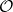over the distinguished open set.

Consider the natural homomorphism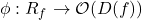which maps a formal fraction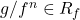to the regular function which is given by the actual quotient of polynomials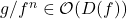. This mapping is straightforwardly injective: suppose that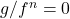on; then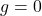onand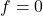on the complement ofby assumption, so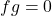everywhere. Hence the preimage of such a functionis exactly 0.

Surjectivity is less trivial. Obviously, every function of the formis regular, but is every function in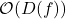globally representable in the form? Yes. Suppose thatis a regular function in. I will sketch out the argument in broad strokes.

First, by the definition of a regular function,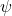is representable as a quotient of polynomials,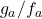, in the neighborhood of every point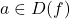. Distinguished open sets are in a sense the “smallest open sets” of the Zariski topology (i.e. they form a basis of the topology), so if we shrink these neighborhoods enough, they become distinguished open sets in their own right, say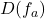for every such point. These open neighborhoods together coverand, conversely, we may say that the variety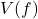is the intersection of every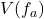or, equivalently, the single variety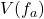.

We have essentially managed to glue together all our little pieces of information about the regular functioninto a single piece of information about, but how can we go further? Translating into the algebraic setting, we know of course that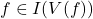, but here we can use the Nullstellensatz to yield a more tractable result; in particular, it tells us that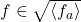, or that(as an element of) is a member of the radical of the ideal which is generated by finitely many elements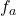.

Now we know that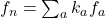for finitely many. Let us define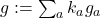, recalling from before that each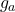comes from the local representation ofasnear. Now taking any arbitrary point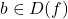with a local representation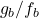of, we can check algebraically that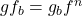and hence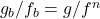. Thereforeis a valid global representation ofover.

February 10th, 2023 | Posted in Math